Question

for the first box, the options are (sp, sp2, sp3) for the second box, the options...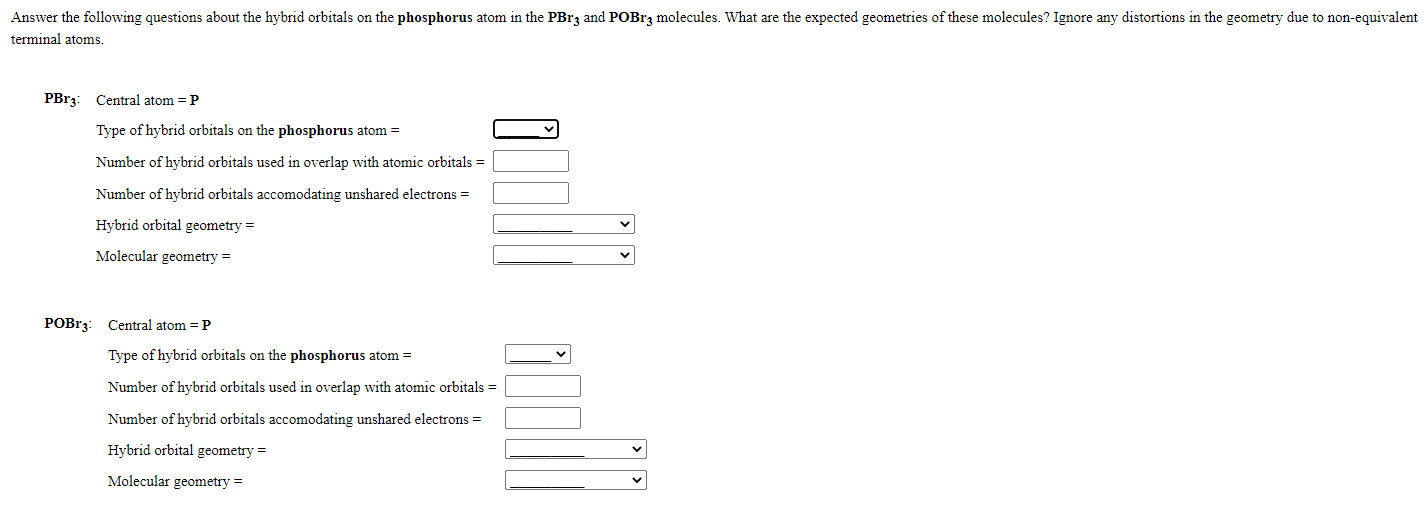for the first box, the options are (sp, sp2, sp3)

for the second box, the options are (linear, bent, trigonal planar, trigonal pyramidal, tetrahedral)

for the third box, the options are (linear, bent, trigonal planar, trigonal pyramidal, tetrahedral)

Answer the following questions about the hybrid orbitals on the phosphorus atom in the PBrz and POBrz molecules. What are the expected geometries of these molecules? Ignore any distortions in the geometry due to non-equivalent terminal atoms. PBrz: Central atom =P Type of hybrid orbitals on the phosphorus atom = Number of hybrid orbitals used in overlap with atomic orbitals = Number of hybrid orbitals accomodating unshared electrons = Hybrid orbital geometry = Molecular geometry = POBrz: Central atom =P Type of hybrid orbitals on the phosphorus atom = Number of hybrid orbitals used in overlap with atomic orbitals = Number of hybrid orbitals accomodating unshared electrons = Hybrid orbital geometry = Molecular geometry =

We need at least 10 more requests to produce the answer.

0 / 10 have requested this problem solution

The more requests, the faster the answer.

All students who have requested the answer will be notified once they are available.

Earn Coins

Coins can be redeemed for fabulous gifts.

Similar Homework Help Questions
• Answer the following questions about the hybrid orbitals on the phosphorus atom in the POCly and...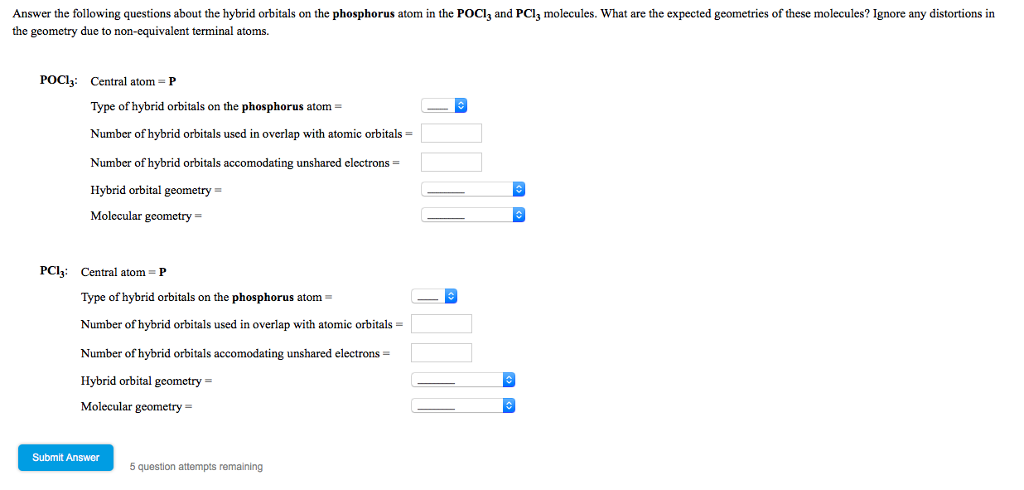Answer the following questions about the hybrid orbitals on the phosphorus atom in the POCly and PCl3 molecules. What are the expected geometries of these molecules? Ignore any distortions in the geometry due to non-equivalent terminal atoms. POCI, Central atom=P Type of hybrid orbitals on the phosphorus atom Number of hybrid orbitals used in overlap with atomic orbitals = Number ofhybrid orbitals accomodating unshared electrons Hybrid orbital geometry Molecular geometry = PC13: Central atom = P Type of hybrid orbitals...

• Consider each of the following molecules and/or ions. Give the hybridization for the central atom in...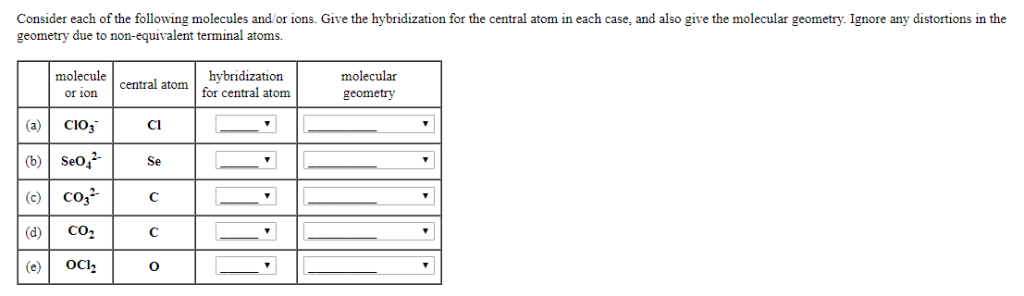Consider each of the following molecules and/or ions. Give the hybridization for the central atom in each case, and also give the molecular geometry. Ignore any distortions in the geometry due to non-equivalent terminal atoms. molecule or ion central atom hybridization for central atom molecular geometry CIO; (b) Seot (c) coza CO2 Cl2 Answer the following questions about the hybrid orbitals on the phosphorus atom in the POCl; and PClz molecules. What are the expected geometries of these molecules? Ignore...

• for the first row of columns (sp,sp2,sp3) for the second row of columns (linear, bent, trigonal...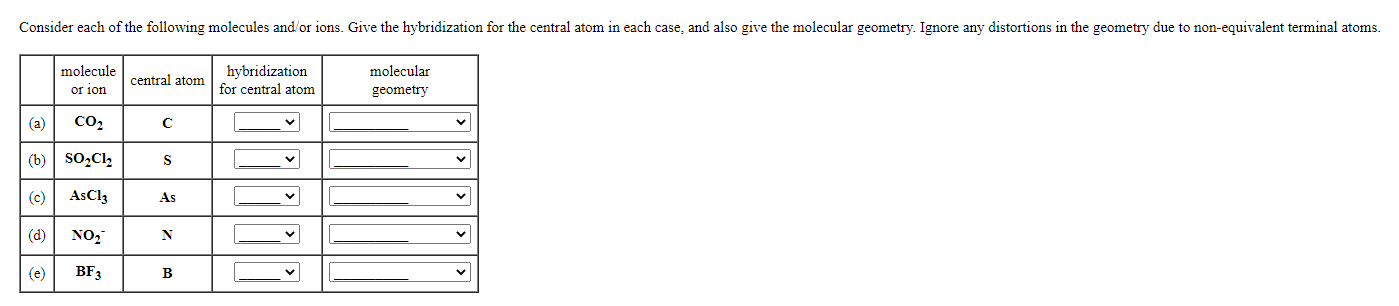for the first row of columns (sp,sp2,sp3) for the second row of columns (linear, bent, trigonal planar, trigonal pyramidal, tetrahedral) Consider each of the following molecules and/or ions. Give the hybridization for the central atom in each case, and also give the molecular geometry. Ignore any distortions in the geometry due to non-equivalent terminal atoms. molecule or ion central atom for central atom hybridization molecular geometry (a) CO2 С (b) SO2Cl2 S (c) AsCl3 As (d) NO2 N (e) BF3...

• Provide the correct molecular geometry for (c), given the number of lone pairs and bonding groups...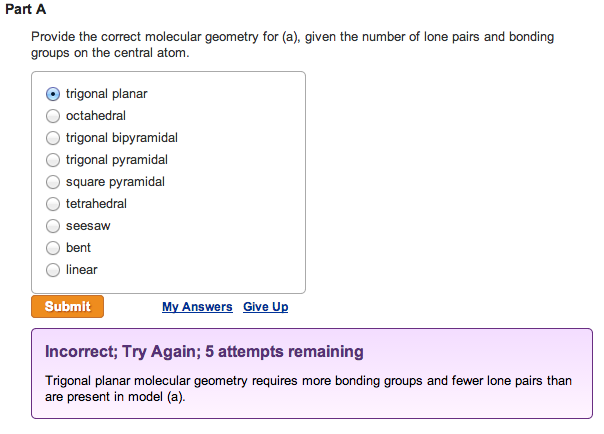Provide the correct molecular geometry for (a), given the number of lone pairs and bonding groups on the central atom. trigonal planar  octahedral  trigonal bipyramidal  trigonal pyramidal  square pyramidal  tetrahedral  seesaw  bent  linearProvide the correct molecular geometry for (b), given the number of lone pairs and bonding groups on the central atom.  trigonal bipyramidal  square pyramidal  bent  tetrahedral  trigonal planar  seesaw  trigonal pyramidal  octahedral  linear Provide the correct molecular geometry for (c), given the number of lone pairs and bonding groups on the...

• Number 1-6 1. What is the molecular geometry of SBr4? A) seesaw B) square planar C)...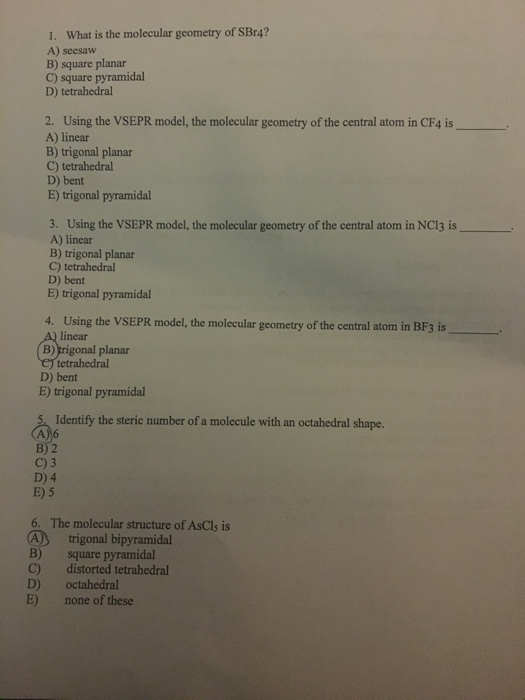Number 1-6 1. What is the molecular geometry of SBr4? A) seesaw B) square planar C) square pyramidal D) tetrahedral 2. Using the VSEPR model, the molecular geometry of the central atom in CF4 is A) linear B) trigonal planar C) tetrahedral D) bent E) trigonal pyramidal 3. Using the VSEPR model, the molecular geometry of the central atom in NC13 is A) linear B) trigonal planar C) tetrahedral D) bent E) trigonal pyramidal 4. Using the VSEPR model, the...

• Please answer correctly I'll give best rating if you do correct one Electron Geomctry Name Choices:...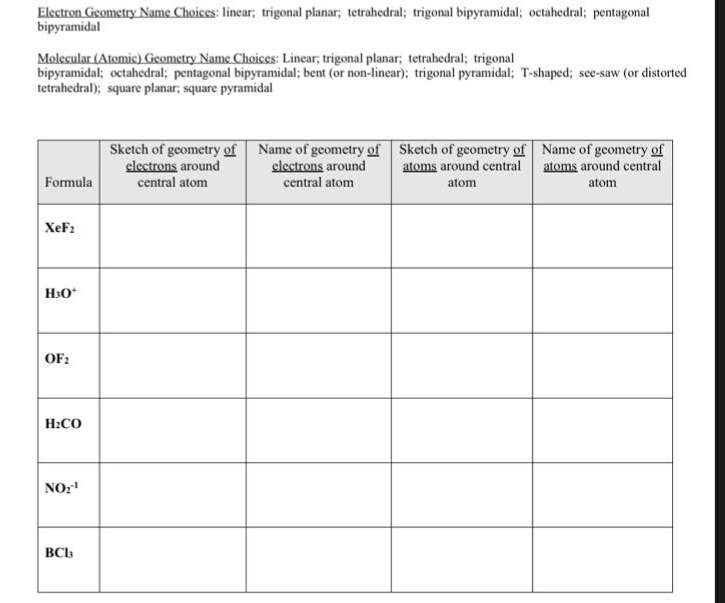Please answer correctly I'll give best rating if you do correct one Electron Geomctry Name Choices: linear; trigonal planar; tetrahedral; trigonal bipyramidal; octahedral; pentagonal bipyramidal Molecular (Atomic) Geometry Name Choices: Linear, trigonal planar; tetrahedral; trigonal bipyramidal; octahedral; pentagonal bipyramidal; bent (or non-linear); trigonal pyramidal: T-shaped; see-saw (or distorted tetrahedral); square planar, square pyramidal Sketch of geometry of Name of geometry of Sketch of geometry of Name of geometry of clectrons around central atom clectrons around atoms around central atoms around...

• VSEPR theory predicts which shape for the molecular structure of the following molecules or molecular ions?...

VSEPR theory predicts which shape for the molecular structure of the following molecules or molecular ions? (i.e. the arrangement of only the bonded atoms about the central atom) bent (or angular) linear octahedral see-saw square planar square pyramidal tetrahedral trigonal bipyramidal trigonal planar trigonal pyramidal T-shaped  ICl3 molecule    (The central atom is I.) bent (or angular) linear octahedral see-saw square planar square pyramidal tetrahedral trigonal bipyramidal trigonal planar trigonal pyramidal T-shaped  IO4- ion    (The central atom is I.) bent (or angular) linear...

• Lewis Dot Structures describe the connectivity of the atoms in a species. a) Determine the centra...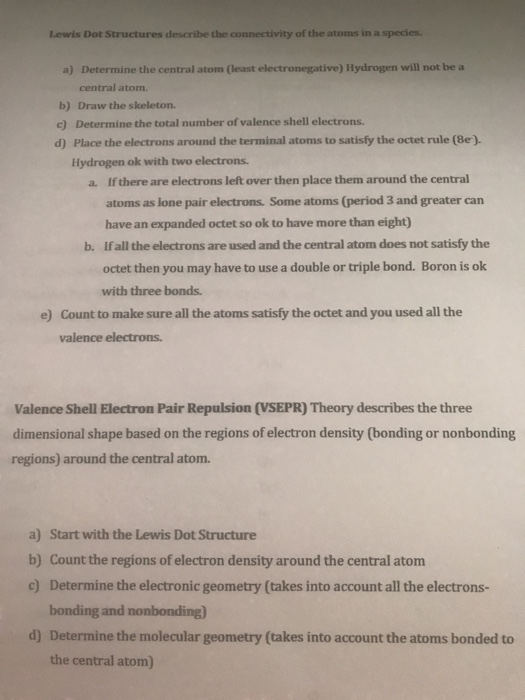Lewis Dot Structures describe the connectivity of the atoms in a species. a) Determine the central atom (least electronegative) Hydrogen will not be a central atom. b) Draw the skeleton. c) Determine the total number of valence shell electrons d) Place the electrons around the terminal atoms to satisfy the octet rule (Be). Hydrogen ok with two electrons. If there are electrons left over then place them around the central atoms as lone pair electrons. Some atoms (period 3 and...

• 2) Use valence bond theory to explain bonding in HCl, and Brci 3) When we mix...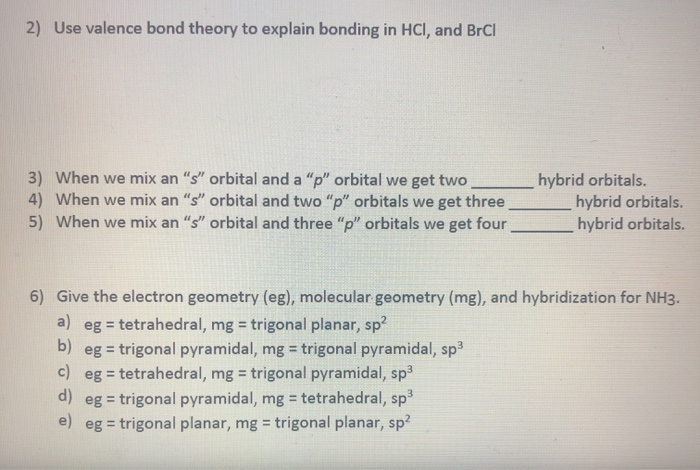2) Use valence bond theory to explain bonding in HCl, and Brci 3) When we mix an "s" orbital and a "p" orbital we get two 4) When we mix an "s" orbital and two "p" orbitals we get three 5) When we mix an "s" orbital and three "p" orbitals we get four hybrid orbitals. hybrid orbitals. hybrid orbitals. 6) Give the electron geometry (eg), molecular geometry (mg), and hybridization for NH3. a) eg = tetrahedral, mg = trigonal...

• (References What is the molecular geometry around an atom that is sp hybridized and has one...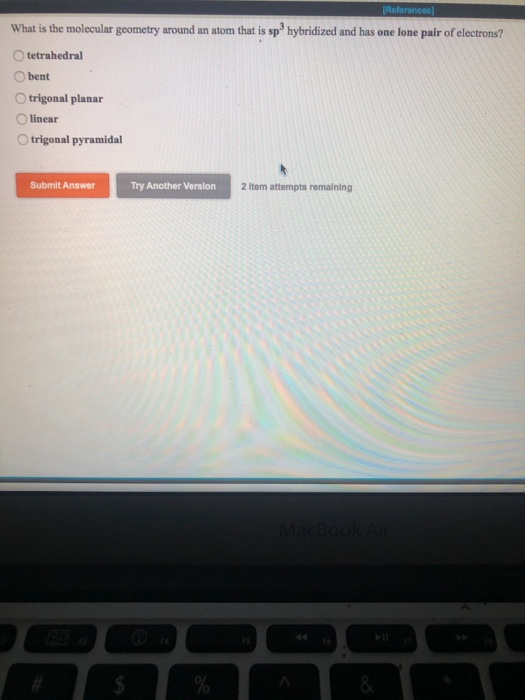(References What is the molecular geometry around an atom that is sp hybridized and has one lone pair of electrons? tetrahedral bent trigonal planar linear trigonal pyramidal Submit Answer Try Another Version 2 item attempts remaining